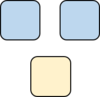# Representing a Context with a Ratio

Alignments to Content Standards: 6.RP.A.1

A recipe calls for 2 cups of tomato sauce and 3 tablespoons of oil. We can say that the ratio of cups of tomato sauce to tablespoons of oil in the recipe is 2:3, or we can say the ratio of tablespoons of oil to cups of tomato sauce is 3:2.For each of the following situations, draw a picture and name two ratios that represent the situation.

1. To make papier-mâché paste, mix 2 parts of water with 1 part of flour.
2. A farm is selling 3 pounds of peaches for \$5.
3. A person walks 6 miles in 2 hours.

## IM Commentary

The purpose of this task is to introduce students to ratios and ratio language.

A teacher might use this task as an opportunity to discuss alternate ways to say a ratio relationship; for example, using the word "to" in place of a colon as shown in the solution.

This task is meant to demonstrate a few nuances to teachers using the task. First, that the order that numbers are written in a ratio does not depend on the order in which things are stated in the question stem. Either order is valid and correctness depends on the words that accompany the numbers as part of a solution. Second, that there is nothing wrong with saying, "The ratio is 6:2." Experienced practioners might show a preference for 3:1, but there's nothing that says this the only correct way to state this ratio relationship. Furthermore, until students have studied what it means for two ratios to be equivalent, they won't understand why we might say the ratio is 3:1. For students who do understand that concept, any equivalent ratio would be acceptable as a solution, but students should be able to explain why if they name ratios that are different than the ones shown in the solution..

The solution shows diagrams that are one step abstracted from literal pictures, which might encourage students to abandon less efficient drawings of more life-like tomatoes, cups, or dollars. The task statement shows different-sized blobs, so that cups are represented with larger blobs and tablespoons are represented with smaller blobs. In the solution, identical units (the cups in part (a)) are drawn as same-sized blobs, and different units (peaches and dollars, miles and hours) are drawn as different-sized blobs. Note that a student might produce a correct drawing where all the blobs are the same size or the relative sizes of the units are not reflected in the diagram. The important thing is that the correct values are shown for each quantity in the ratio (3 blobs and 5 blobs to represent a peach to dollar ratio of 3:5, for example.) Also, a student may have learned previously to use a different representation such as a tape diagram, and a solution using a different type of representation is perfectly acceptable. The solution shown does not presume students have knowledge of more sophisticated types of diagrams.

## Solution

1. The ratio of cups of water to cups of flour is 2:1. The ratio of cups of flour to cups of water is 1:2.2. The ratio of peaches to dollars is 3:5. The ratio of dollars to peaches is 5:3.3. The ratio of miles to hours is 6:2. The ratio of hours to miles is 2:6.